How to Calculate and Solve for Compressional or P Waves | Rock Mechanics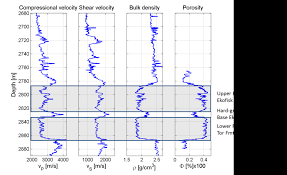The image above represents compressional or P waves.

To compute for compressional or P waves, three essential parameters are needed and these parameters are Dynamic Bulk Modulus of Elasticity (kd), Dynamic Modulus of Rigidity (Gdand Elasticity of Rock Mass (p).

The formula for calculating compressional or P waves:

vp = √[kd + 4/3Gd] / p

Where:

vp = Compressional or P Waves
kd = Dynamic Bulk Modulus of Elasticity
Gd = Dynamic Modulus of Rigidity
p = Elasticity of the Rock Mass

Let’s solve an example;
Given that the dynamic bulk modulus of elasticity is 11, the dynamic modulus of rigidity is 30 and the elasticity of the rock mass is 23. Find the compressional or P waves?

This implies that;

kd = Dynamic Bulk Modulus of Elasticity = 11
Gd = Dynamic Modulus of Rigidity = 30
p = Elasticity of the Rock Mass = 23

vp = √[kd + 4/3Gd] / p
vp = √[11 + 4/3(30)] / 23
vp = √[11 + 40] / 23
vp = √ / 23
vp = 7.141 / 23
vp = 0.310

Therefore, the compressional or P waves is 0.310.

How to Calculate and Solve for Shear Force or S Waves | Rock Mechanics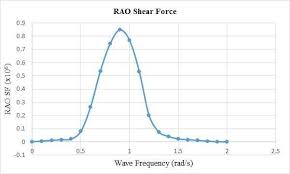The image above represents shear force or S waves.

To compute for shear force or S waves, two essential parameters are needed and these parameters are Dynamic Modulus of Rigidity (Gd) and Elasticity of Rock Mass (p).

The formula for calculating shear force or S waves:

vs = √(Gd) / p

Where:

vs = Shear Force or S Waves
Gd = Dynamic Modulus of Rigidity
p = Elasticity of Rock Mass

Let’s solve an example;
Find the shear force or s waves when the dynamic modulus of rigidity is 12 and the elasticity of rock mass is 28.

This implies that;

Gd = Dynamic Modulus of Rigidity = 12
p = Elasticity of Rock Mass = 28

vs = √(Gd) / p
vs = √(12) / 28
vs = 3.46 / 28
vs = 0.12

Therefore, the shear force or S waves is 0.12.

Calculating the Dynamic Modulus of Rigidity when the Shear Force or S Waves and the Elasticity of Rock Mass is Given.

Gd = (vs x p)2

Where:

Gd = Dynamic Modulus of Rigidity
vs = Shear Force or S Waves
p = Elasticity of Rock Mass

Let’s solve an example;
Find the dynamic modulus of rigidity when the shear force or s waves is 21 and the elasticity of rock mass is 14.

This implies that;

vs = Shear Force or S Waves = 21
p = Elasticity of Rock Mass = 14

Gd = (vs x p)2
Gd = (21 x 14)2
Gd = (294)2
Gd = 86436

Therefore, the dynamic modulus of rigidity is 86436.

How to Calculate and Solve for Rock Density Designation | Rock Mechanics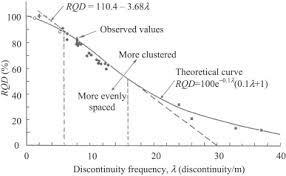The image above represents rock quality designation.

To compute for rock quality designation, two essential parameters are needed and these parameters are Sum of the Length of Core Pieces > 10cm (S) and Total Length Drilled or Core Recovery (L).

The formula for calculating the rock quality designation:

RQD = 100(S / L)

Where:

RQD = Rock Quality Designation
S = Sum of the Length of Core Pieces > 10cm
L = Total Length Drilled or Core Recovery

Let’s solve an example;
Calculate the rock quality designation? Given that the sum of the length of core pieces > 10cm is 20 and the total length drilled or core recovery is 14.

This implies that;

S = Sum of the Length of Core Pieces > 10cm = 20
L = Total Length Drilled or Core Recovery = 14

RQD = 100(S / L)
RQD = 100(20 / 14)
RQD = 100(1.428)
RQD = 142.85

Therefore, the rock quality designation is 142.85.

Calculating the Sum of the Length of Core Pieces > 10cm when the Rock Quality Designation and the Total Length Drilled or Core Recovery is Given.

S = RQD x L

Where:

S = Sum of the Length of Core Pieces > 10cm
RQD = Rock Quality Designation
L = Total Length Drilled or Core Recovery

Let’s solve an example;
Calculate the sum of the length of core pieces > 10cm? Given that the rock quality designation is 32 and the total length drilled or core recovery is 11.

This implies that;

RQD = Rock Quality Designation = 32
L = Total Length Drilled or Core Recovery = 11

S = RQD x L
S = 32 x 11
S = 352

Therefore, the sum of the length of core pieces > 10cm is 352.

How to Calculate and Solve for Bulk Modulus of Elasticity (E) | Rock Mechanics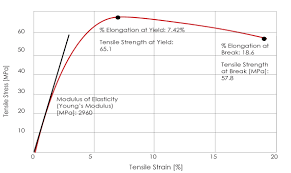The image above represents bulk modulus of elasticity (E).

To compute for bulk modulus of elasticity (E), two essential parameters are needed and these parameters are young’s modulus (E) and Poisson’s ratio (v).

The formula for calculating the bulk modulus of elasticity (E):

k = E / 3(1 – 2v)

Where:

k = Bulk Modulus of Elasticity (E)
E = Young’s Modulus
v = Poisson’s Ratio

Let’s solve an example;
Find the bulk modulus of elasticity (E) when the young’s modulus is 66 and the Poisson’s ratio is 58.

This implies that;

E = Young’s Modulus = 66
v = Poisson’s Ratio = 58

k = E / 3(1 – 2v)
k = 66 / 3(1 – 2(58))
k = 66 / 3(1 – 116)
k = 66 / 3(-115)
k = 66 / -345
k = -0.19

Therefore, the bulk modulus of elasticity (E) is -0.19.

Calculating the Young’s Modulus when the Bulk Modulus of Elasticity (E) and the Poisson’s Ratio is Given.

E = k (3 – 6v)

Where:

E = Young’s Modulus
k = Bulk Modulus of Elasticity (E)
v = Poisson’s Ratio

Let’s solve an example;
Find the young’s modulus when the bulk modulus of elasticity (E) is 22 and the Poisson’s ratio is 18.

This implies that;

k = Bulk Modulus of Elasticity (E) = 22
v = Poisson’s Ratio = 18

E = k (3 – 6v)
E = 22 (3 – 6(18))
E = 22 (3 – 108)
E = 22 (-105)
E = -2376

Therefore, the young’s modulus is -2376.

How to Calculate and Solve for Mohr – Coulomb Criterion | Rock Mechanics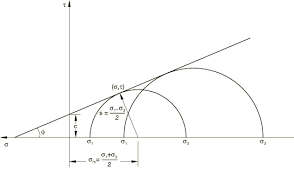The image above represents mohr – coulomb criterion.

To compute for mohr – coulomb criterion, three essential parameters are needed and these parameters are Cohesion (|τo), Co-efficient of Friction (μ) and Normal Stress (σn).

The formula for calculating the mohr – coulomb criterion:

|τ| = τo + μσn

Where;

|τ = Mohr – Coulomb Criterion
o = Cohesion
μ = Co-efficient of Friction
σn = Normal Stress

Let’s solve an example;
Find the mohr – coulomb criterion when the cohesion is 7, the co-efficient of friction is 30 and the normal stress is 28.

This implies that;

o = Cohesion = 7
μ = Co-efficient of Friction = 30
σn = Normal Stress = 28

|τ| = τo + μσn
|τ| = 7 + 30(28)
|τ| = 7 + 840
|τ| = 847

Therefore, the mohr – coulomb criterion is 847.

Calculating the Cohesion when the Mohr – Coulomb Criterion, the Co-efficient of Friction and the Normal Stress is Given.

o = |τ|  – μσn

Where;

o = Cohesion
|τ = Mohr – Coulomb Criterion
μ = Co-efficient of Friction
σn = Normal Stress

Let’s solve an example;
Find the cohesion when the mohr – coulomb criterion is 44, the co-efficient of friction is 10 and the normal stress is 4.

This implies that;

|τ = Mohr – Coulomb Criterion = 44
μ = Co-efficient of Friction = 10
σn = Normal Stress = 4

o = |τ|  – μσn
o = 44  – 10(4)
o = 44  – 40
o = 4

Therefore, the cohesion is 4.

How to Calculate and Solve for Friction Angle | Rock Mechanics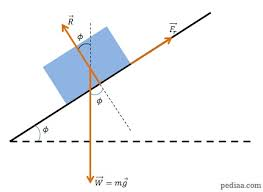The image above represents friction angle.

To compute for friction angle, two essential parameters are needed and these parameters are Uniaxial Compressive Strength (σc) and cohesion (c).

The formula for calculating the friction angle:

φ = 2tan-1(σc – 2c / σc + 2c)

Where;

φ = Friction Angle
σc = Uniaxial Compressive Strength
c = Cohesion

Let’s solve an example;
Find the friction angle when the uniaxial compressive strength is 11 and the cohesion is 22.

This implies that;

σc = Uniaxial Compressive Strength = 11
c = Cohesion = 22

φ = 2tan-1(σc – 2c / σc + 2c)
φ = 2tan-1(11 – 2(22) / 11 + 2(22))
φ = 2tan-1(11 – 44 / 11 + 44)
φ = 2tan-1(-33 / 55)
φ = 2tan-1(-0.6)
φ = 2(-30.96°)
φ = -61.927

Therefore,  the friction angle is -61.927.

How to Calculate and Solve for Shear Modulus | Rock Mechanics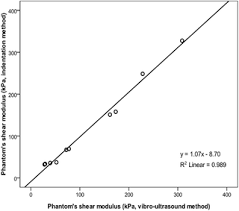The image above represents shear modulus.

To compute for shear modulus, two essential parameters are needed and these parameters are young’s modulus (E) and Poisson’s ratio (v).

The formula for calculating the shear modulus:

G = E / 2(1 + v)

Where:

G = Shear Modulus
E = Young’s Modulus
v = Poisson’s Ratio

Let’s solve an example;
Find the shear modulus when the young’s modulus is 32 and the Poisson’s ratio is 24.

This implies that;

E = Young’s Modulus = 32
v = Poisson’s Ratio = 24

G = E / 2(1 + v)
G = 32 / 2(1 + 24)
G = 32 / 2(25)
G = 32 / 50
G = 0.64

Therefore, the shear modulus is 0.64.

Calculating the Young’s Modulus when the Shear Modulus and the Poisson’s Ratio is Given.

E = G (2 + 2v)

Where:

E = Young’s Modulus
G = Shear Modulus
v = Poisson’s Ratio

Let’s solve an example;
Find the young’s modulus when the shear modulus is 12 and the Poisson’s ratio is 10.

This implies that;

G = Shear Modulus = 12
v = Poisson’s Ratio = 10

E = G (2 +2v)
E = 12 (2 + 2(10))
E = 12 (2 + 20)
E = 12 (22)
E = 264

Therefore, the young’s modulus is 264.

How to Calculate and Solve for Bulk Modulus of Elasticity (stress) | Rock Mechanics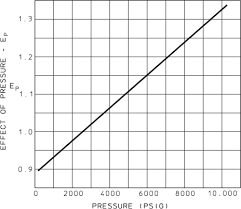The image above represents bulk modulus of elasticity (stress).

To compute for bulk modulus elasticity (stress), two essential parameters are needed and these parameters are Average Horizontal Stress (hav) and Vertical Stress (σv).

The formula for calculating the bulk modulus elasticity (stress):

k = hav / σv

Where;

k = Bulk Modulus of Elasticity (Stress)
hav = Average Horizontal Stress
σv = Vertical Stress

Let’s solve an example;
Given that the average horizontal stress is 15 and the vertical stress is 34. Find the bulk modulus of elasticity (stress)?

This implies that;

hav = Average Horizontal Stress = 15
σv = Vertical Stress = 34

k = hav / σv
k = 15 / 34
k = 0.44

Therefore, the bulk modulus of elasticity (stress) is 0.44.

Calculating the Average Horizontal Stress when the Bulk Modulus Elasticity (stress) and the Vertical Stress is Given.

hav = k x σv

Where;

hav = Average Horizontal Stress
k = Bulk Modulus of Elasticity (Stress)
σv = Vertical Stress

Let’s solve an example;
Given that the bulk modulus of elasticity (stress) is 25 and the vertical stress is 8. Find the average horizontal stress?

This implies that;

k = Bulk Modulus of Elasticity (Stress) = 25
σv = Vertical Stress = 8

hav = k x σv
hav = 25 x 8
hav = 200

Therefore, the average horizontal stress is 200.

How to Calculate and Solve for Horizontal Strain | Rock Mechanics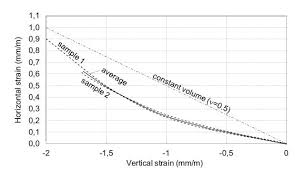The image above represents horizontal strain.

To compute for horizontal strain, five essential parameters are needed and these parameters are Principal Horizontal Stress Component 1 (σH1), Principal Horizontal Stress Component 2 (σH2), Poisson’s Ratio (v), Vertical Stress (σv) and Young’s Modulus (E).

The formula for calculating the horizontal strain:

εH = H1 – vσH2 – vσv) / E

Where:

εH = Horizontal Strain
σH1 = Principal Horizontal Stress Component 1
σH2 = Principal Horizontal Stress Component 2
v = Poisson’s Ratio
σv = Vertical Stress
E = Young’s Modulus

Let’s solve an example;
Find the horizontal strain when the principal horizontal stress component 1 is 7, the principal horizontal component 2 is 9, the Poisson’s ratio is 11, the vertical stress is 21 and young’s modulus is 27.

This implies that;

σH1 = Principal Horizontal Stress Component 1 = 7
σH2 = Principal Horizontal Stress Component 2 = 9
v = Poisson’s Ratio = 11
σv = Vertical Stress = 21
E = Young’s Modulus = 27

εH = H1 – vσH2 – vσv) / E
εH = (7 – 11(9) – 11(21)) / 27
εH = (7 – 99 – 231) / 27
εH = -323 / 27
εH = -11.96

Therefore, the horizontal strain is -11.96.

Calculating the Principal Horizontal Stress Component 1 when the Horizontal Stress, Principal Horizontal Stress Component 2, Poisson’s Ratio, Vertical Stress and Young’s Modulus is Given.

σH1 = (εH x E) + vσH2 + vσv

Where;

σH1 = Principal Horizontal Stress Component 1
εH = Horizontal Strain
σH2 = Principal Horizontal Stress Component 2
v = Poisson’s Ratio
σv = Vertical Stress
E = Young’s Modulus

Let’s solve an example;
Find the principal horizontal stress component 1 when the horizontal strain is 22, the principal horizontal component 2 is 10, the Poisson’s ratio is 7, the vertical stress is 12 and young’s modulus is 3.

This implies that;

εH = Horizontal Strain = 22
σH2 = Principal Horizontal Stress Component 2 = 10
v = Poisson’s Ratio = 7
σv = Vertical Stress = 12
E = Young’s Modulus = 3

σH1 = (εH x E) + vσH2 + vσv
σH1 = (22 x 3) + 7(10) + 7(12)
σH1 = 66 + 70 + 84
σH1 = 220

Therefore, the principal horizontal stress component 1 is 220.

Calculating the Principal Horizontal Stress Component 2 when the Horizontal Stress, Principal Horizontal Stress Component 1, Poisson’s Ratio, Vertical Stress and Young’s Modulus is Given.

σH2 = -(H x E) – σH1 + vσv / v)

Where;

σH2 = Principal Horizontal Stress Component 2
εH = Horizontal Strain
σH1 = Principal Horizontal Stress Component 1
v = Poisson’s Ratio
σv = Vertical Stress
E = Young’s Modulus

Let’s solve an example;
Find the principal horizontal stress component 2 when the horizontal strain is 28, the principal horizontal component 1 is 14, the Poisson’s ratio is 5, the vertical stress is 17 and young’s modulus is 7.

This implies that;

εH = Horizontal Strain =28
σH1 = Principal Horizontal Stress Component 1 = 14
v = Poisson’s Ratio = 5
σv = Vertical Stress = 17
E = Young’s Modulus = 7

σH2 = -(H x E) – σH1 + vσv / v)
σH2 = -((28 x 7) – 14 + 5(17) / 5)
σH2 = -(196 – 14 + 85 / 5)
σH2 = -(182 + 85 / 5)
σH2 = -(267 / 5)
σH2 = -(53.4)
σH2 = -53.4

Therefore, the principal horizontal stress component 2 is -53.4.

How to Calculate and Solve for Poisson’s Ratio | Rock Mechanics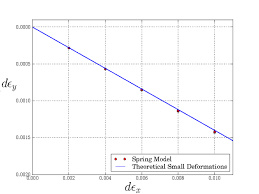The image above represents Poisson’s ratio.

To compute for Poisson’s ratio, two essential parameters are needed and these parameters are lateral strain (εland axial strain (εa).

The formula for calculating Poisson’s ratio:

v = εl / εa

Where:

v = Poisson’s Ratio
εl = Lateral Strain
εa = Axial Strain

Let’s solve an example;
Find the Poisson’s ratio when the lateral strain is 80 and the axial strain is 4.

This implies that;

εl = Lateral Strain = 80
εa = Axial Strain = 4

v = εl / εa
v = 80 / 4
v = 20

Therefore, the Poisson’s ratio is 20.

Calculating the Lateral Strain when the Poisson’s Ratio and Axial Strain is Given.

εl = v x εa

Where:

εl = Lateral Strain
v = Poisson’s Ratio
εa = Axial Strain

Let’s solve an example;
Find the lateral strain when the Poisson’s ratio is 40 and the axial strain is 9.

This implies that;

v = Poisson’s Ratio = 40
εa = Axial Strain = 9

εl = v x εa
εl = 40 x 9
εl = 360

Therefore, the lateral strain is 360.